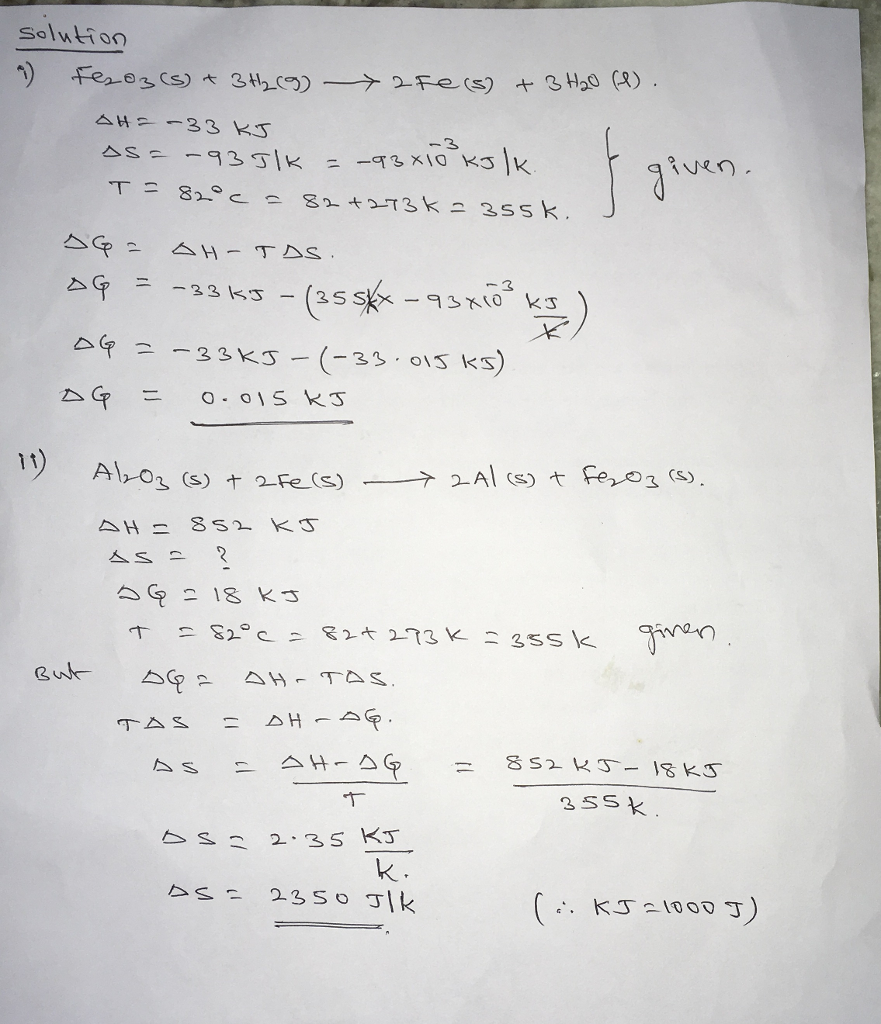# Homework Solution: Sponta because the system is at equilibrium. Fe_2O_3(s) + 3H_2(g) rightarrow…

Sponta because the system is at equilibrium. Fe_2O_3(s) + 3H_2(g) rightarrow 2Fe(s) + 3H_2O(l) delta H = -33 kJ delta S = -93 J/K delta G = kJ Which is spontaneous? this reaction the reverse reaction neither Al_2O_3(s) + 2Fe(s) rightarrow 2Al(s) + Fe_2O_3(s) delta H = 852 kJ delta S = J/K delta G = 18 kJ Which is spontaneous? this reaction the reverse reaction neither